International
Tables for
Crystallography
Volume D
Physical properties of crystals
Edited by A. Authier

International Tables for Crystallography (2006). Vol. D, ch. 1.1, pp. 8-9

## Section 1.1.3.5. Representation surface of a tensor

A. Authiera*

aInstitut de Minéralogie et de la Physique des Milieux Condensés, Bâtiment 7, 140 rue de Lourmel, 75015 Paris, France
Correspondence e-mail: aauthier@wanadoo.fr

| top | pdf |

#### 1.1.3.5.1. Definition

| top | pdf |

Let us consider a tensor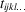represented in an orthonormal frame where variance is not important. The value of component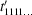in an arbitrary direction is given by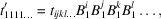where the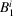,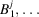are the direction cosines of that direction with respect to the axes of the orthonormal frame.

The representation surface of the tensor is the polar plot of.

#### 1.1.3.5.2. Representation surfaces of second-rank tensors

| top | pdf |

The representation surfaces of second-rank tensors are quadrics. The directions of their principal axes are obtained as follows. Let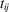be a second-rank tensor and let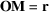be a vector with coordinates. The doubly contracted product,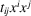, is a scalar. The locus of points M such that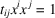is a quadric. Its principal axes are along the directions of the eigenvectors of the matrix with elements. They are solutions of the set of equations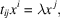where the associated quantities λ are the eigenvalues.

Let us take as axes the principal axes. The equation of the quadric reduces to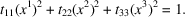If the eigenvalues are all of the same sign, the quadric is an ellipsoid; if two are positive and one is negative, the quadric is a hyperboloid with one sheet; if one is positive and two are negative, the quadric is a hyperboloid with two sheets (see Section 1.3.1).

Associated quadrics are very useful for the geometric representation of physical properties characterized by a tensor of rank 2, as shown by the following examples:

 (i) Index of refraction of a medium. It is related to the dielectric constant by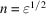and, like it, it is a tensor of rank 2. Its associated quadric is an ellipsoid, the optical indicatrix, which represents its variations with the direction in space (see Section 1.6.3.2). (ii) Thermal expansion. If one cuts a sphere in a medium whose thermal expansion is anisotropic, and if one changes the temperature, the sphere becomes an ellipsoid. Thermal expansion is therefore represented by a tensor of rank 2 (see Chapter 1.4). (iii) Thermal conductivity. Let us place a drop of wax on a plate of gypsum, and then apply a hot point at the centre. There appears a halo where the wax has melted: it is elliptical, indicating anisotropic conduction. Thermal conductivity is represented by a tensor of rank 2 and the elliptical halo of molten wax corresponds to the intersection of the associated ellipsoid with the plane of the plate of gypsum.

#### 1.1.3.5.3. Representation surfaces of higher-rank tensors

| top | pdf |

Examples of representation surfaces of higher-rank tensors are given in Sections 1.3.3.4.4and 1.9.4.2.